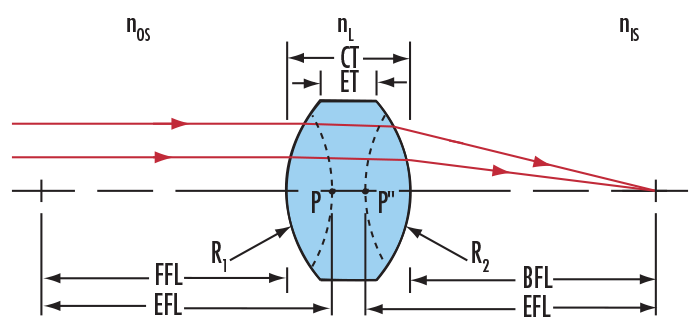# Focal Length Calculator

View All Technical Tools

### Center Thickness, CT (mm):

Effective Focal Length, EFL (mm): --

Back Focal Length, BFL (mm): --

Front Focal Length, FFL (mm): --

Primary Principal Point, P (mm): --

Secondary Principal Point, P'' (mm): --

Shift in Nodal Point (mm): >--$$\Phi_{\text{OS}} = \frac{n_L - n_{\text{OS}}}{R_1}$$
$$\Phi_{\text{IS}} = \frac{ n_{\text{IS}} - n_{L} }{R_2}$$
$$\Phi = \Phi_{\text{OS}} + \Phi_{\text{IS}} - \Phi_{\text{OS}} \, \Phi_{\text{IS}} \, \left( \frac{\text{CT}}{n_L} \right)$$
$$P'' = - \frac{\Phi_{\text{OS}}}{\Phi} \, \left( \frac{n_{\text{IS}}}{n_L} \right) \, \text{CT}$$
$$\text{EFL} = \frac{1}{\Phi}$$
$$f_F = - n_{\text{OS}} \cdot \text{EFL}$$
$$f_R = n_{\text{IS}} \cdot \text{EFL}$$
$$\text{BFL} = f_R + P''$$
$$\text{FFL} = f_F + P$$
$$\text{NPS} = f_F + f_R$$
$$P = \frac{\Phi_{\text{IS}}}{\Phi} \, \left( \frac{n_{\text{OS}}}{n_L} \right) \, \text{CT}$$
 ΦOS Power of Surface 1 ΦIS Power of Surface 2 F Lens Power nOS Object Space Index nL Lens Index nIS Image Space Index R1 Radius of Surface 1 R2 Radius of Surface 2 CT Center Thickness
 P Primary Principle Point P" Secondary Principle Point EFL Effective Focal Length BFL Back Focal Length FFL Front Focal Length NPS Nodal Point Shift fF Front Focal Point fR Rear Focal Point

Note: This calculator follows the standard sign convention for the optical radius of curvature where if the vertex of a surface lies to the left of the center of curvature the radius of curvature is positive, and if the vertex lies to the right of the center of curvature the radius of curvature is negative. In the figure, R1 is positive and R2 is negative.

## Description

Relate optical and mechanical parameters of simple lenses in order to ease integration into application assemblies. Select index from list of EO's own optical substrates to help calculate focal lengths and principal points of any standard lens.

### Application Note

Understanding Focal Length and Field of View